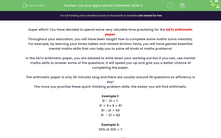# Use and Apply Mental Arithmetic Skills 3

In this worksheet, students will practise foundational mental maths skills in preparation for the SATs arithmetic paper. This worksheet will guide them through specific strategies enabling them to solve problems mentally.This content is premium and exclusive to EdPlace subscribers.Key stage:  KS 2

Curriculum topic:   Exam-style Questions: SATs Maths

Curriculum subtopic:   Exam-Style Questions: Mental Maths

Difficulty level:#### Worksheet Overview

Super effort! You have decided to spend some very valuable time practising for the SATs arithmetic paper.

Throughout your education, you will have been taught how to complete some maths calculations mentally.

For example, by learning your times tables and related division facts, you will have gained essential mental maths skills that can help you to solve all kinds of maths problems!

In the SATs arithmetic paper, you are allowed to write down your working out but if you can, use mental maths skills to answer some of the questions, it will speed you up and give you a better chance of completing the paper.

The arithmetic paper is only 30 minutes long and there are usually around 36 questions, so efficiency is key!

The more you practise these quick-thinking problem skills, the easier you will find arithmetic.

Example 1:

9² - 21 = ?

9² = 9 x 9 = 81

81 - 21 = 60

9²  - 21 = 60

Example 2:

93% of 320 = ?

Step 1: Work out 90%

10% = 320 ÷ 10 = 32

90% = 32 x 9 = 288

Step 2: To find 3%, find 1% first

1% = 320 ÷ 100 = 3.2

3% = 3.2 x 3 = 9.6

Step 3: Add 90% + 3%

288 + 9.6 = 297.6

93% of 320 = 297.6

Over to you now to try a mixture of quick-fire arithmetic questions!

### What is EdPlace?

We're your National Curriculum aligned online education content provider helping each child succeed in English, maths and science from year 1 to GCSE. With an EdPlace account you’ll be able to track and measure progress, helping each child achieve their best. We build confidence and attainment by personalising each child’s learning at a level that suits them.

Get started